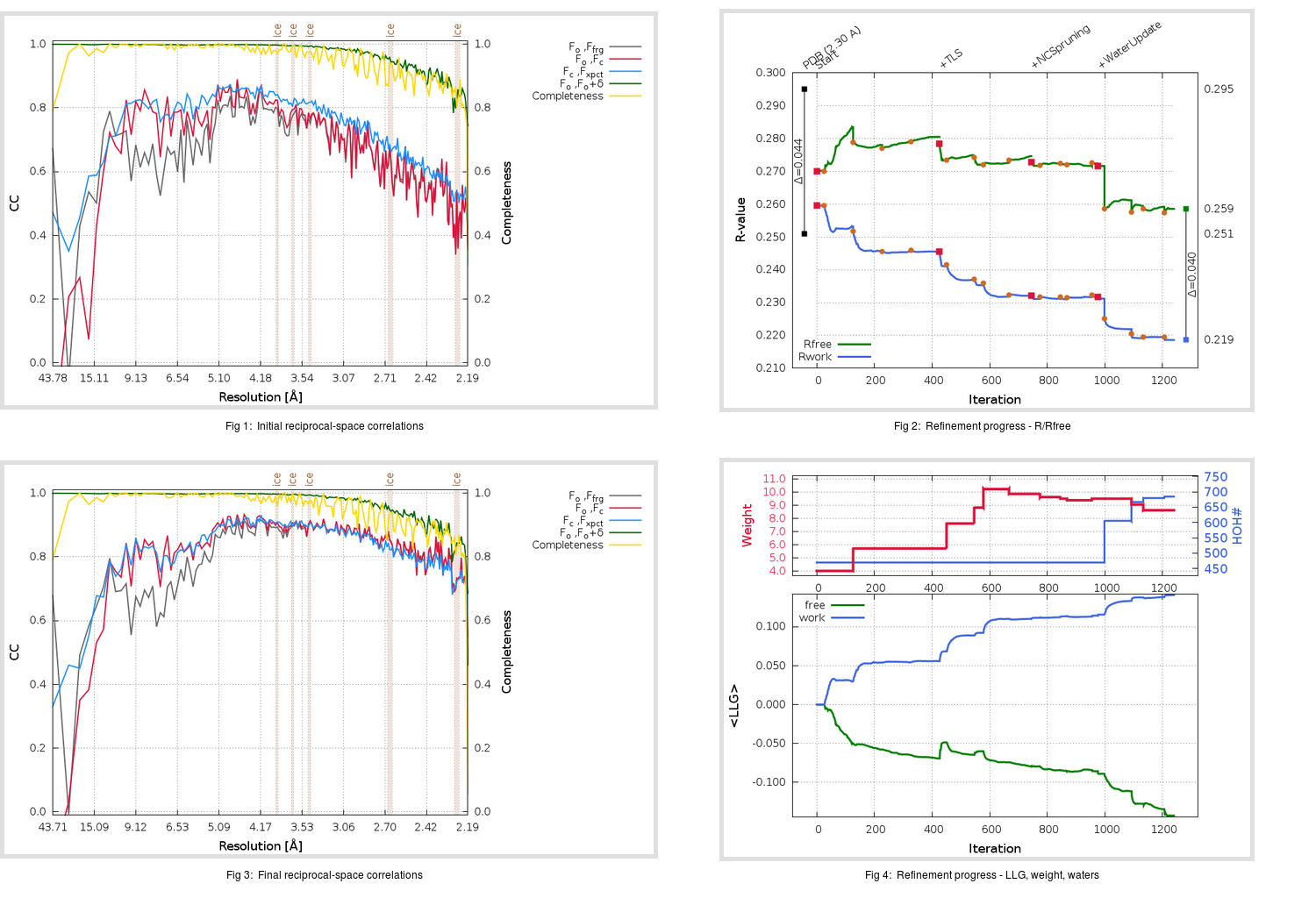Content:

```    Diffraction limits & principal axes of ellipsoid fitted to diffraction cut-off surface:
2.113         0.9978   0.0000  -0.0664       0.969 a* - 0.248 c*
2.070         0.0000   1.0000   0.0000       b*
2.661         0.0664   0.0000   0.9978       0.047 a* + 0.999 c*
```

## Deposited

` `
 Date deposited Date data collection Resolution R, Rfree 20060327 20050920 2.30 0.2480 0.2950

Molprobity (CCP4 7.0 version) summary:

```Ramachandran outliers =   0.48 %
favored =  93.25 %
Rotamer outliers      =   3.48 %
C-beta deviations     =     1
Clashscore            =   7.79
RMS(bonds)            =   0.0088
RMS(angles)           =   1.21
MolProbity score      =   2.27
Resolution            =   2.30
R-work                =   0.2480
R-free                =   0.2950
```

```Number of waters      =   470

<B> (all atoms) =   20.95 ( sd =    4.80 ) for       7089 non-hydrogen atoms
<B>   (protein) =   21.15 ( sd =    4.30 ) for       6614 non-hydrogen atoms
<B>     (water) =   18.19 ( sd =    8.88 ) for        470 non-hydrogen atoms
<B>    (others) =   11.04 ( sd =    4.71 ) for          5 non-hydrogen atoms

B min/max       (all non-hydrogen atoms) =    2.00 /   48.47
B min/max   (protein non-hydrogen atoms) =    7.17 /   45.26
B min/max     (water non-hydrogen atoms) =    2.00 /   48.47
B min/max     (other non-hydrogen atoms) =    3.92 /   17.23
```

## BUSTER (re-)refinement

` `

Molprobity (CCP4 7.0 version) summary:

```Ramachandran outliers =   0.48 %
favored =  94.22 %
Rotamer outliers      =   5.01 %
C-beta deviations     =     0
Clashscore            =   5.17
RMS(bonds)            =   0.0115
RMS(angles)           =   1.65
MolProbity score      =   2.20
Resolution            =   2.19
R-work                =   0.2186
R-free                =   0.2586
```

```Number of waters      =   685

<B> (all atoms) =   31.57 ( sd =   12.28 ) for       7304 non-hydrogen atoms
<B>   (protein) =   30.93 ( sd =   12.00 ) for       6614 non-hydrogen atoms
<B>     (water) =   37.50 ( sd =   13.04 ) for        685 non-hydrogen atoms
<B>    (others) =   61.06 ( sd =   13.77 ) for          5 non-hydrogen atoms

B min/max       (all non-hydrogen atoms) =    9.21 /   92.99
B min/max   (protein non-hydrogen atoms) =   11.99 /   92.99
B min/max     (water non-hydrogen atoms) =    9.21 /   92.30
B min/max     (other non-hydrogen atoms) =   48.30 /   86.30
```

Refinement progression:Results:

` `
 File Remark 2GHW_aB_refine.01_04_refine.pdb.gz exact refinement commands are in header 2GHW_aB_refine.01_04_refine.mtz.gz including original deposited data and several re-refinement map coefficients 2GHW_aB_refine.01_04_BUSTER_model.cif.gz including any non-standard compound restraints 2GHW_aB_refine.01_04_BUSTER_refln.cif.gz